# buck ,DC to Dc converter

#### Veracohr

Joined Jan 3, 2011
767
Look closely at the diagram on the right. The negative side of VL is the same as the positive side of Vout, and the negative side of Vout is the same as the positive side of VL. Therefore, VL=-Vout.

•micro1

#### BramLabs

Joined Nov 21, 2013
98
That's negative because it's negativeSorry if my picture is pain in the '*ss' :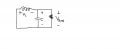or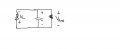As you can see on the picture above, when the switch or transistor is in 'OFF' condition, the voltage on the inductor, will be opposite to the output voltage.

And remember, that inductor has a characteristic to 'maintain' the current. So it means that when inductor charge and discharged (current), it has different polarity of CURRENT, not the VOLTAGE. So basically, from charge (+) (-) can change into discharge (-)(+)
And it's only happened to the inductor, not the capacitor. Capacitor won't change the polarity of the current. But inductor does change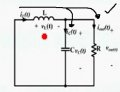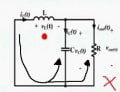Last edited:
•micro1

#### MrAl

Joined Jun 17, 2014
9,781
Hi,

hi
buck ,DC to Dc converter
why is there negave with Vout?

Vl(t) = - Vout(t)

View attachment 103648

The second diagram is incorrect because it shows the polarity of the inductor the same as it was before the field started to collapse.
Once the field starts to collapse, the voltage reverses and thus charges the capacitor positively.

So when the transistor turns off, the diode conducts and the voltage across the inductor changes from +L- to -L+, with the positive on the right hand side now. That's the way inductors behave in circuits like this.

•micro1

#### BramLabs

Joined Nov 21, 2013
98
Hi,

The second diagram is incorrect because it shows the polarity of the inductor the same as it was before the field started to collapse.
Once the field starts to collapse, the voltage reverses and thus charges the capacitor positively.

So when the transistor turns off, the diode conducts and the voltage across the inductor changes from +L- to -L+, with the positive on the right hand side now. That's the way inductors behave in circuits like this.
I guess you have the wrong idea sir. The voltage of inductor doesn't change, but the 'current' itself should be change. And from the picture that TS posted, it's written that vL(t)=-Vout(t) , and the picture shown the vL(t), not the iL(t) polarity. In case of your answer is for iL(t), but in the picture TS has shown above, it's for showing vL(t)
And i completely agree with that. The voltage DOES NOT change the polarity, but the current DOES (because the characteristic of inductor). It's a little bit confusing i guess at the start, but once i learned, it's more interesting•micro1

#### anhnha

Joined Apr 19, 2012
901
Hi,

The second diagram is incorrect because it shows the polarity of the inductor the same as it was before the field started to collapse.
Once the field starts to collapse, the voltage reverses and thus charges the capacitor positively.

So when the transistor turns off, the diode conducts and the voltage across the inductor changes from +L- to -L+, with the positive on the right hand side now. That's the way inductors behave in circuits like this.
I think you made a mistake here. In the second diagram, the polarity of inductor voltage is what we can choose, it can be arbitrary, no matter what it is in reality.
If the result is positive, then the chosen polarity is correct, otherwise, it is the other way around.

#### MrAl

Joined Jun 17, 2014
9,781
Hello again BramLabs, anhnha,

I guess you have the wrong idea sir. The voltage of inductor doesn't change, but the 'current' itself should be change. And from the picture that TS posted, it's written that vL(t)=-Vout(t) , and the picture shown the vL(t), not the iL(t) polarity. In case of your answer is for iL(t), but in the picture TS has shown above, it's for showing vL(t)
And i completely agree with that. The voltage DOES NOT change the polarity, but the current DOES (because the characteristic of inductor). It's a little bit confusing i guess at the start, but once i learned, it's more interestingI am not sure where you are getting that information from, but once the transistor turns off the inductor must switch voltage polarity. If that did not happen, the diode would not be able to conduct. In other words, if you are right then show how the diode can conduct with a positive voltage at it's cathode with say 5v across the capacitor (as in a 10v to 5v buck converter).

I think you made a mistake here. In the second diagram, the polarity of inductor voltage is what we can choose, it can be arbitrary, no matter what it is in reality.
If the result is positive, then the chosen polarity is correct, otherwise, it is the other way around.
Are we talking about the same circuit?

In the buck circuit, when the transistor is on the current flows from input to output through the inductor as the current rises, and the capacitor charges a little.
When the transistor starts to turn off, the left side of the inductor is positive and the right side is less positive. However, after a short time the transistor resistance climbs fast, and as it gets higher the current in the inductor starts to decrease. The decrease in current would cause the inductor voltage to flip polarity, and therefore the diode conducts. The inductor then dumps some of its energy into the capacitor.

How do i know the inductor voltage changes polarity?
One way is to use the unsigned version definition of an inductor:
v=L*di/dt

when the current is increasing di/dt>0, so v must be positive because L times a positive number yields a positive voltage.
When the current is decreasing di/dt<0, so v must be negative because L times a negative number yields a negative voltage.

The average current can be left to right all the time yet the CHANGE in that current may go plus and minus, which it does.

Another way is to look at the field. When the current is increasing the field is increasing, and the polarity is one way.
When the current is decreasing the field is decreasing, and as we all know the back EMF creates an opposite polarity voltage (like a generator).

If you dont agree, then show how the diode can conduct when the transistor turns off during an average cycle. This should also show that the polarity can not be 'arbitrary' and not sure why anyone would think it can be.

If i did not understand your question or explanation properly, please feel free to elaborate.

•#### BramLabs

Joined Nov 21, 2013
98
Hello again BramLabs, anhnha,

I am not sure where you are getting that information from, but once the transistor turns off the inductor must switch voltage polarity. If that did not happen, the diode would not be able to conduct. In other words, if you are right then show how the diode can conduct with a positive voltage at it's cathode with say 5v across the capacitor (as in a 10v to 5v buck converter).
Nahhh, that's the tricky part. I'm completely agree with you.
In your case, let's say :
Vinput = 10V
and you want :
Voutput = 5V

It means that, we should dissipate 5V at the inductor.
So in the easy ways, it should be :

10V (input) - 5V (inductor) = 5V (load)
Then we get the load 5V as you mentioned before.

And let's talk about the polarity.
Input 10V --> (+) at top, and (-) at bottom or ground.
And if the inductor it's in (-) at the left of the inductor and (+) at the right of the inductor as you have been said before (off state switch), because you said that the voltage polarity change, then if we applied KVL, it would be 10V + 5V, then we get = 15V
Is that a boost converter or buck converter ?
The idea of buck converter is to 'decrease' the voltage using inductor right ?
So in simple way is we just need to have a different polarity between 10V and 5V, so we can get either 5V or 15V depends on the polarity of the supply and the polarity of inductor.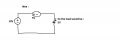And then based on the idea, we change the 5V voltage as an inductor.
And if we applied the formula :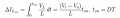From the picture :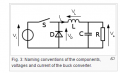In this case, 10V (input) - 5V (output).
And we get vL = Vi - Vo
And how's the polarity ?
If we subtitute from the equation above, we get :
Vi - vL = Vo
Then we should have a different polarity so that they aren't adding each other. But they have to substract each other to make 10V(input) - 5V(inductor) = 5V (load)

Then we'll get the answerFor your diode question, let me think for a while. ahahhaha...(kind of busy). I'll answer it laterOr maybe if someone has proved that you're right, i'll correct myself and learn from it ^^

Last edited:

Joined Nov 5, 2010
215
I'm with MrAl on this. Voltage in a circuit is not arbitrary. It is clearly defined by physics.

Here is another way to look at it: the role of the inductor switches from a passive, driven component to an active one that supplies the voltage.

During the ON phase of the transistor, the voltage source drives a current through the inductor into the capacitor. The leading edge of the inductor is as positive as the supply voltage - transistor voltage drop, and because of the inductor's impedance, its trailing edge must be more negative.

During the OFF phase of the transistor, the collapsing field induces a current in the inductor. In order for this current to flow, the trailing edge of the inductor flips to positive and the leading edge flips to negative because the inductor BECOMES the voltage source. And as MrAl points out, the only way current will flow in the transistor OFF phase is to turn on the diode, which requires the trailing edge of the inductor to become more positive than the charge on the capacitor plus the voltage drop of the diode. This is the only way the diode will switch on.

Here is an AAC article about induced voltage that includes the diagram below, showing the voltage flip on the inductor. Note the arrows are showing electron flow instead of classic current flow, but the voltage polarity is the same.#### anhnha

Joined Apr 19, 2012
901
The second diagram is incorrect because it shows the polarity of the inductor the same as it was before the field started to collapse.
Once the field starts to collapse, the voltage reverses and thus charges the capacitor positively.
About the actual polarity of inductor, you are right. However, I just disagree with what you said here that the the second diagram is incorrect.
The polarity of inductor voltage is what we choose before solving the circuit. The actual polarity will be known after getting the value.
For example, with the circuit above, we choose the left end is plus and the right end of inductor is minus.
When the switch is off we have the picture on the right.
In this case, VL = -Vout < 0. So the actual polarity of inductor voltage is just reverse what we assume initally.
I would disagree with saying that the second diagram is wrong here.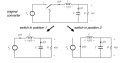Joined Nov 5, 2010
215
It means that, we should dissipate 5V at the inductor.
So in the easy ways, it should be :

10V (input) - 5V (inductor) = 5V (load)
Then we get the load 5V as you mentioned before.
Well, yes, during the transistor ON phase the inductor is more + to the left and - to the right, as a function of its current flow and impedance creating a voltage drop.

And let's talk about the polarity.
Input 10V --> (+) at top, and (-) at bottom or ground.
And if the inductor it's in (-) at the left of the inductor and (+) at the right of the inductor as you have been said before (off state switch), because you said that the voltage polarity change, then if we applied KVL, it would be 10V + 5V, then we get = 15V
Wait a minute, are you talking about the OFF state or the ON state? In the transistor OFF state the 10V is no longer there. The only voltage source present is the induced voltage in the inductor - on the left and + on the right, which drives against the voltage on the capacitor to deposit a little more charge on it (by flowing current through the diode).

In this case, 10V (input) - 5V (output).
And we get vL = Vi - Vo
And how's the polarity ?
If we subtitute from the equation above, we get :
Vi - vL = Vo
Then we should have a different polarity so that they aren't adding each other. But they have to substract each other to make 10V(input) - 5V(inductor) = 5V (load)
I'm not sure what you're trying to say here, but the formulas are reasonable, but only as they apply to the diagram, and the formulas are ONLY talking about the transistor ON phase. They show that during the ON phase, the inductor voltage is + on the left and - on the right.

When the switch opens the 10V is no longer there and the collapsing field on the inductor turns it into a voltage supply with - on the left and + on the right.

I think your problem may be that you're mixing up the ON phase and the OFF phase.

#### BramLabs

Joined Nov 21, 2013
98
I'm with MrAl on this. Voltage in a circuit is not arbitrary. It is clearly defined by physics.

Here is another way to look at it: the role of the inductor switches from a passive, driven component to an active one that supplies the voltage.

During the ON phase of the transistor, the voltage source drives a current through the inductor into the capacitor. The leading edge of the inductor is as positive as the supply voltage - transistor voltage drop, and because of the inductor's impedance, its trailing edge must be more negative.

During the OFF phase of the transistor, the collapsing field induces a current in the inductor. In order for this current to flow, the trailing edge of the inductor flips to positive and the leading edge flips to negative because the inductor BECOMES the voltage source. And as MrAl points out, the only way current will flow in the transistor OFF phase is to turn on the diode, which requires the trailing edge of the inductor to become more positive than the charge on the capacitor plus the voltage drop of the diode. This is the only way the diode will switch on.

Here is an AAC article about induced voltage that includes the diagram below, showing the voltage flip on the inductor. Note the arrows are showing electron flow instead of classic current flow, but the voltage polarity is the same."because the inductor BECOMES the voltage source" that's the part when you're wrong sir.
Inductor doesn't act as an voltage source, but it acts as an current source sir when chargedAnd capacitor is the one who becomes a voltage source when charged^^
An ideal "voltage source" is simply an element providing voltage across its output that DOES NOT depend upon the current flowing, for the "current source" it is vice versa.

I'm completely agree with sir anhnha

The question for TS is that why is there negative Vout.
The answer is just by the look on the picture ^^

Well, yes, during the transistor ON phase the inductor is more + to the left and - to the right, as a function of its current flow and impedance creating a voltage drop.

Wait a minute, are you talking about the OFF state or the ON state? In the transistor OFF state the 10V is no longer there. The only voltage source present is the induced voltage in the inductor - on the left and + on the right, which drives against the voltage on the capacitor to deposit a little more charge on it (by flowing current through the diode).

I'm not sure what you're trying to say here, but the formulas are reasonable, but only as they apply to the diagram, and the formulas are ONLY talking about the transistor ON phase. They show that during the ON phase, the inductor voltage is + on the left and - on the right.

When the switch opens the 10V is no longer there and the collapsing field on the inductor turns it into a voltage supply with - on the left and + on the right.

I think your problem may be that you're mixing up the ON phase and the OFF phase.
I think my explanation wasn't good at all. Sure, we can't mix ON phase to the OFF phase, but we can't definitely only say when it's OFF phase or it's ON phase. We should analyze it when OFF state FIRST and then ON state. Not only OFF state or ON stateBecause if we only analyze it when it's in ON state, we couldn't see the way why it has 'negative' value of Vout.
If you say that when it's ON state, the the inductor switch the 'voltage' polarity, then this website ( https://en.wikipedia.org/wiki/Buck_converter ) shows a wrong calculation and explanation.

In my opinion, inductor switch the 'current' polarity by its voltage. BUT the voltage of inductor itself, doesn't changeIt's confusing isn't it ? ahahahaha ^^

Last edited:

#### Jony130

Joined Feb 17, 2009
5,439
vL = 1/L integral vL dt
What ??
The voltage across an ideal inductor is VL = L* ΔI/Δt
This means that to produce the voltage across an inductance, the applied current must change. If the current is kept constant, no voltage will be induced, no matter how large the current. Conversely, if it is found that the voltage across an inductance is zero this means that the current must be constant but not necessary zero.
So in BUCK convert the voltage across the inductor changes his polarity. And here you have the proof
I build this circuit on the breadboard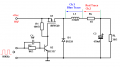And for F = 10KHz I set the duty to 56% to obtain 5V output at 39Ω load.
I also measure voltage across the inductor and use Rs resistor to measure inductor current.

The blue trace shows the voltage across the inductor. As you can see the voltage across the coil changes his polarity.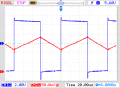When the switch is ON the voltage across the inductor is equal to VL ≈ +4.8V. But when the switch is OFF (D1 ON) the voltage is VL ≈ - 6V.
And this voltage must be negative because the inductor current ramp-down (as we can see on the read trace) and this means that the rate of change (ΔI/Δt) is negative, so the voltage also must be negative.
Or we can view it like this:
The goal of the inductor is to resist changes in current flow.
During the ON-time the upper end of the inductor gets set to a higher voltage than its lower end. And the current flow from left to right.
But when the switch opens (OFF), the input DC source gets disconnected from the inductor. But the inductor wants to keep the current to flow in the same direction as it was when the switch was closed (from left to right). And this can only happen when the inductor change his polarity (the inductor becomes a sort of “voltage source" now). And thanks to this the current will keep on flowing in the SAME direction during the switch OFF as it was flowing before. And this is why we get the apparent sign reversal for voltage.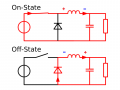However in fact voltage reversal does not always occur . That is because the primary requirement is only that the
inductor current somehow needs to keep flowing in the SAME direction.

Last edited:

Joined Nov 5, 2010
215
About the actual polarity of inductor, you are right. However, I just disagree with what you said here that the the second diagram is incorrect.
The polarity of inductor voltage is what we choose before solving the circuit. The actual polarity will be known after getting the value.
For example, with the circuit above, we choose the left end is plus and the right end of inductor is minus.
When the switch is off we have the picture on the right.
In this case, VL = -Vout < 0. So the actual polarity of inductor voltage is just reverse what we assume initally.
I would disagree with saying that the second diagram is wrong here.

View attachment 103711
OK, the second diagram isn't "wrong" IF YOU TAKE IT IN CONTEXT. It doesn't show the polarity of the voltage on the inductor. It shows the polarity CONVENTION of their equations. If you read on from those diagrams, they explain that in their text. The +/- labeling in the diagrams is simply a convention to explain the polarities in their equations. And during the OFF phase, the drawing and equations below show that the polarity reverses and becomes - to the left and + to the right, the negative of what they show in the drawing above. -v(t) means that the voltage during the OFF phase is the reverse of the labeled convention.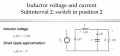Joined Nov 5, 2010
215
"because the inductor BECOMES the voltage source" that's the part when you're wrong sir.
Inductor doesn't act as an voltage source, but it acts as an current source sir when chargedAnd capacitor is the one who becomes a voltage source when charged^^
If the inductor is a current source it is also a voltage source. No current will flow in the circuit unless the right end of the inductor is more + than the charged voltage on the capacitor.

#### MrAl

Joined Jun 17, 2014
9,781
Hello again BramLabs and InspectorGadget and Anhnha,

Ok, i see now why there is confusion over the polarity, however, we must also take into account the wording of the first post in this thread which implies that it is the static polarity that is in question, not the as yet undetermined polarity. So to be true to the first post i believe that i had interpreted the meaning of the OP correctly, even though the pure meaning, had we had more in depth information to begin with, would be as Anhnha has explained. So we are all talking about two different interpretations of the same drawing, and i will explain each view and how it applies in just a minute.

Anhnha:
Ok, i see what you are saying now, and i believe you are correct insofar as your assumptions. However, the OP apparently took the other view where the polarities are ALL post polarities, as many people would. It's only when we see a theoretical paper that chooses to draw the circuit that way do we see the true intent, which is the pre-analysis polarities. You also might want to note that BramLabs is agreeing with you even though he is viewing this as having the post polarities drawnJony has also now shown the correct post polarities.

Others:
Yes, we have either the pre-polarity interpretation or the post polarity interpretation. My own descriptions came from viewing these as post polarities, where the polarities for each mode are shown independently.

BramLabs:
You agree with anhnha but your drawings show that you are still insisting that the inductor does not change voltage.
For the post polarity view, the voltage LEVEL is arbitrary but the voltage POLARITY is distinct, and it changes sign.

The pre-polarity interpretation applies to the theoretical equation writing process, while the post polarity interpretation applies to the actual voltage during the mode in question.
However, EITHER of these interpretations will eventually show that the actual inductor voltage changes polarity in the actual circuit.

•#### BramLabs

Joined Nov 21, 2013
98
What ??
The voltage across an ideal inductor is VL = L* ΔI/Δt
This means that to produce the voltage across an inductance, the applied current must change. If the current is kept constant, no voltage will be induced, no matter how large the current. Conversely, if it is found that the voltage across an inductance is zero this means that the current must be constant but not necessary zero.
So in BUCK convert the voltage across the inductor changes his polarity. And here you have the proof
I build this circuit on the breadboard
View attachment 103707

And for F = 10KHz I set the duty to 56% to obtain 5V output at 39Ω load.
I also measure voltage across the inductor and use Rs resistor to measure inductor current.

The blue trace shows the voltage across the inductor. As you can see the voltage across the coil changes his polarity.
View attachment 103708

When the switch is ON the voltage across the inductor is equal to VL ≈ +4.8V. But when the switch is OFF (D1 ON) the voltage is VL ≈ - 6V.
And this voltage must be negative because the inductor current ramp-down (as we can see on the read trace) and this is why the rare of change (ΔI/Δt) is negative so the voltage also must be negative.
Or we can view this way:
The goal of the inductor is to resist changes in current flow.
During the ON-time the upper end of the inductor gets set to a higher voltage than its lower end. And the current flow from left to right.
But when the switch opens (OFF), the input DC source gets disconnected from the inductor. But the inductor wants to keep the current to flow in the same direction as it was when the switch was closed (from left to right). And this can only happen when the inductor change his polarity (the inductor becomes a sort of “voltage source" now). And thanks to this the current will keep on flowing in the SAME direction during the switch OFF as it was flowing before. And this is why we get the apparent sign reversal for voltage.
View attachment 103712
However in fact voltage reversal does not always occur . That is because the primary requirement is only that the
inductor current somehow needs to keep flowing in the SAME direction.
Oh, i already fixed it sir. ahahahah

Joined Nov 5, 2010
215
What ??
The voltage across an ideal inductor is VL = L* ΔI/Δt
This means that to produce the voltage across an inductance, the applied current must change. If the current is kept constant, no voltage will be induced, no matter how large the current.
I agree.

Conversely, if it is found that the voltage across an inductance is zero this means that the current must be constant but not necessary zero.
If the voltage across the inductor is zero, no current will flow, because the voltage at the right end of the inductor needs to be greater than the voltage on the capacitor and the voltage on the left end of the inductor needs to be more negative than the voltage drop of the diode in order for current to flow.

So in BUCK convert the voltage across the inductor changes his polarity. And here you have the proof
I build this circuit on the breadboard
View attachment 103707

And for F = 10KHz I set the duty to 56% to obtain 5V output at 39Ω load.
I also measure voltage across the inductor and use Rs resistor to measure inductor current.

The blue trace shows the voltage across the inductor. As you can see the voltage across the coil changes his polarity.
View attachment 103708

When the switch is ON the voltage is across the inductor is equal to VL ≈ +4.8V. But when the switch is OFF (D1 ON) the voltage is VL ≈ - 6V.
And this voltage must be negative because the inductor current ramp-down (as we can see on the read trace) and this is why the rare of change (ΔI/Δt) is negative so the voltage also must be negative.
Or we can view this way:
The goal of the inductor is to resist changes in current flow.
During the ON-time the upper end of the inductor gets set to a higher voltage than its lower end. And the current flow from left to right.
But when the switch opens (OFF), the input DC source gets disconnected from the inductor. But the inductor wants to keep the current to flow in the same direction as it was when the switch was closed (from left to right). And this can only happen when the inductor change his polarity (the inductor becomes a sort of “voltage source" now). And thanks to this the current will keep on flowing in the SAME direction during the switch OFF as it was flowing before. And this is why we get the apparent sign reversal for voltage.
View attachment 103712
However in fact voltage reversal does not always occur . That is because the primary requirement is only that the
inductor current somehow needs to keep flowing in the SAME direction.
This is all true, except for the fact that, as your simulation and diagrams say, there must be a polarity switch on the inductor, as it goes from a passive component in phase 1 to an active component in phase 2.

Otherwise, where is the voltage, the "electromotive force," to drive the current? No, it is NOT the voltage on the capacitor. This is the output, this is what another voltage must drive against in order to replace the charge drained off by the load.

In order for the "inductor current ... to keep flowing in the SAME direction" there must be a voltage source to drive the circuit. In phase 2, the transistor OFF phase, this voltage comes from the inductor itself, driven by the induced current.

A current cannot keep flowing if there is no voltage source to drive that current.

#### BramLabs

Joined Nov 21, 2013
98
OK, the second diagram isn't "wrong" IF YOU TAKE IT IN CONTEXT. It doesn't show the polarity of the voltage on the inductor. It shows the polarity CONVENTION of their equations. If you read on from those diagrams, they explain that in their text. The +/- labeling in the diagrams is simply a convention to explain the polarities in their equations. And during the OFF phase, the drawing and equations below show that the polarity reverses and becomes - to the left and + to the right, the negative of what they show in the drawing above. -v(t) means that the voltage during the OFF phase is the reverse of the labeled convention.

View attachment 103713
Woah, now it's looked more confusing. ahahahaha...
Okay now i'm agree with the term of the polarity change.
Thank you for the explanation sir.

The hardest part i think, when we (students), got a question and at the 'question' already defined the polarity of the inductor, and when we want to analyze it, and we should mark the polarity, my mind would be like, "Oh sh!t, my lecturer made a joke of us. If i marked polarity of the inductor like on the question, then i will have a wrong answer in reality. But if i followed the polarity as in the question, then i got the wrong anaylze of buck converter".
No one ever got 100 in the class then. ahahahaha

Last edited:

#### Jony130

Joined Feb 17, 2009
5,439